# Fill in the Blanks: Solutions | JEE Advanced Notes | Study Chemistry 35 Years JEE Main & Advanced Past year Papers - JEE

## JEE: Fill in the Blanks: Solutions | JEE Advanced Notes | Study Chemistry 35 Years JEE Main & Advanced Past year Papers - JEE

The document Fill in the Blanks: Solutions | JEE Advanced Notes | Study Chemistry 35 Years JEE Main & Advanced Past year Papers - JEE is a part of the JEE Course Chemistry 35 Years JEE Main & Advanced Past year Papers.
All you need of JEE at this link: JEE

Fill in the Blanks

1. Given that ΔTf is the depression in freezing point of the solvent in a solution of a non-volatile solute of molality, m, the quantity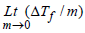is equal to ........... .       (1994 - 1 Mark)

Ans: Kf

Solutions : Kf; Depression in freezing point, ΔTf = Kf . m, where Kf is the molar depression constant or cryoscopic constant and m is the molality of the solution given by moles of solute per 1000 g of the solvent.

Integer Value Correct Type

1. 29.2% (w/w) HCl stock solution has a density of 1.25 g mL–1. The molecular weight of HCl is 36.5 g mol–1. The volume (mL) of stock solution required to prepare a 200 mL
solution of 0.4 M HCl is :     (2012)

Ans : 8

Solution:  Molarity of stock solution of HCl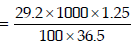Let the volume of stock solution required = V mL
Thus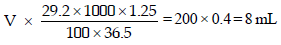2. MX2 dissociates into M2+ and X ions in an aqueous solution, with a degree of dissociation (a) of 0.5. The ratio of the observed depression of freezing point of the aqueous solution to the value of the depression of freezing point in the absence of ionic dissociation is (JEE Adv. 2014)

Ans : 2

Solutions: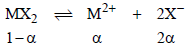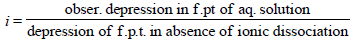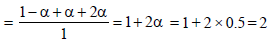3. A compound H2X with molar weight of 80g is dissolved in a solvent having density of 0.4 g ml–1. Assuming no change in volume upon dissolution, the molality of a 3.2 molar solution is (JEE Adv. 2014)

Ans : 8

Solutions :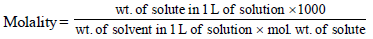Calculation of wt. of solvent
1 mL of solvent = 0.4 g
1000 mL of solvent = 400 g
Calculation of wt. of solute
1000 mL of solution contain = 3.2 × 80 g solute = 256 g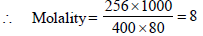4. If the freezing point of a 0.01 molal aqueous solution of a cobalt(III) chloride-ammonia complex (which behaves as a strong electrolyte) is –0.0558°C, the number of chloride(s) in the coordination sphere of the complex is [Kf of water = 1.86 K kg mol–1] (JEE Adv. 2015)

Ans : 1

Solution :

Given ΔTf = 0.0558°C
as we know, ΔTf = i × Kf × m
⇒ 0.0558 = i × 1.86 × 0.01
i = 3

Therefore the complex will be [Co(NH3)5Cl]Cl2
Hence number of chloride in co-ordination sphere is 1.

5. The mole fraction of a solute in a solution is 0.1. At 298 K, molarity of this solution is the same as its molality. Density of this solution at 298 K is 2.0 g cm–3 . The ratio of the molecular weights of the solute and solvent,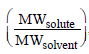, is

Ans : 9

solution : 1 mole solution has 0.1 mole solute and 0.9 mole solvent.
Let M1 = Molar mass solute
M2 = Molar mass solvent

Molality, m =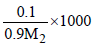.....(1)

Molarity, M =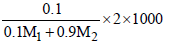.......(2)

∵  m = M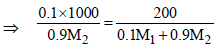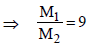The document Fill in the Blanks: Solutions | JEE Advanced Notes | Study Chemistry 35 Years JEE Main & Advanced Past year Papers - JEE is a part of the JEE Course Chemistry 35 Years JEE Main & Advanced Past year Papers.
All you need of JEE at this link: JEEUse Code STAYHOME200 and get INR 200 additional OFF

## Chemistry 35 Years JEE Main & Advanced Past year Papers

122 docs|66 tests

Track your progress, build streaks, highlight & save important lessons and more!

,

,

,

,

,

,

,

,

,

,

,

,

,

,

,

,

,

,

,

,

,

;